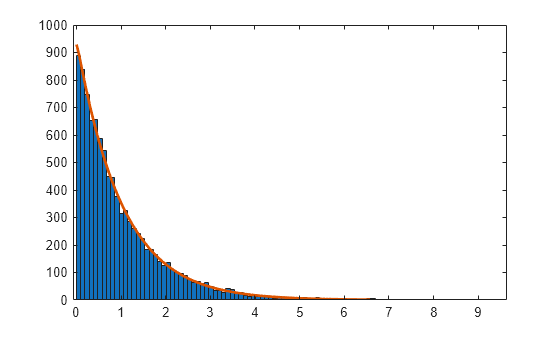# random

## 语法

``R = random(name,A)``
``R = random(name,A,B)``
``R = random(name,A,B,C)``
``R = random(name,A,B,C,D)``
``R = random(pd)``
``R = random(___,sz1,...,szN)``
``R = random(___,sz)``

## 说明

``R = random(name,A)` 从由 `name` 和分布参数 `A` 指定的单参数分布族中返回一个随机数。`

``R = random(name,A,B)` 从由 `name` 和分布参数 `A` 和 `B` 指定的双参数分布族中返回一个随机数。`
``R = random(name,A,B,C)` 从由 `name` 和分布参数 `A`、`B` 和 `C` 指定的三参数分布族中返回一个随机数。`
``R = random(name,A,B,C,D)` 从由 `name` 和分布参数 `A`、`B`、`C` 和 `D` 指定的四参数分布族中返回一个随机数。`

``R = random(pd)` 从概率分布对象 `pd` 中返回一个随机数。`

``R = random(___,sz1,...,szN)` 使用上述任一语法的输入参数，根据指定的概率分布生成一个随机数数组，其中 `sz1,...,szN` 表示每个维度的大小。`

``R = random(___,sz)` 使用上述任一语法的输入参数，根据指定的概率分布生成一个随机数数组，其中向量 `sz` 指定 `size(r)`。`

## 示例

```rng('default') % For reproducibility mu = 1; sigma = 5; r = random('Normal',mu,sigma)```
```r = 3.6883 ```

```mu = 1; sigma = 5; pd = makedist('Normal','mu',mu,'sigma',sigma);```

```rng('default') % For reproducibility r = random(pd)```
```r = 3.6883 ```

```s = rng; r = random('Poisson',5)```
```r = 5 ```

```rng(s); r1 = random('Poisson',5)```
```r1 = 5 ```

```A = [3 2; -2 1]; sz = size(A); R = random('Stable',2,0,1,0,sz)```
```R = 2×2 0.7604 -3.1945 2.5935 1.2193 ```

`R = random('Stable',2,0,1,0,size(A))`
```R = 2×2 0.4508 -0.6132 -1.8494 0.4845 ```

`pd = makedist('Weibull')`
```pd = WeibullDistribution Weibull distribution A = 1 B = 1 ```

```rng('default') % For reproducibility r = random(pd,10000,1);```

`histfit(r,100,'weibull')``pd = makedist('Normal')`
```pd = NormalDistribution Normal distribution mu = 0 sigma = 1 ```

`r = random(pd,[2,3,2])`
```r = r(:,:,1) = 0.5377 -2.2588 0.3188 1.8339 0.8622 -1.3077 r(:,:,2) = -0.4336 3.5784 -1.3499 0.3426 2.7694 3.0349 ```

## 输入参数

`name`分布输入参数 `A`输入参数 `B`输入参数 `C`输入参数 `D`
`'Beta'`Beta Distributiona 第一个形状参数b 第二个形状参数
`'Binomial'`Binomial Distributionn 试验次数p 每次试验成功的概率
`'BirnbaumSaunders'`Birnbaum-Saunders Distributionβ 尺度参数γ 形状参数
`'Burr'`Burr Type XII Distributionα 尺度参数c 第一个形状参数k 第二个形状参数
`'Chisquare'``'chi2'`Chi-Square Distributionν 自由度
`'Exponential'`Exponential Distributionμ 均值
`'Extreme Value'``'ev'`Extreme Value Distributionμ 位置参数σ 尺度参数
`'F'`F Distributionν1 分子自由度ν2 分母自由度
`'Gamma'`Gamma Distributiona 形状参数b 尺度参数
`'Generalized Extreme Value'``'gev'`Generalized Extreme Value Distributionk 形状参数σ 尺度参数μ 位置参数
`'Generalized Pareto'``'gp'`Generalized Pareto Distributionk 尾部指数（形状）参数σ 尺度参数μ 阈值（位置）参数
`'Geometric'`Geometric Distributionp 概率参数
`'Half Normal'``'hn'`Half-Normal Distributionμ 位置参数σ 尺度参数
`'Hypergeometric'``'hyge'`Hypergeometric Distributionm 总体的大小k 总体中具有所需特征的项数n 抽取的样本数量
`'InverseGaussian'`Inverse Gaussian Distributionμ 尺度参数λ 形状参数
`'Logistic'`逻辑分布μ 均值σ 尺度参数
`'LogLogistic'`Loglogistic Distributionμ 对数值的均值σ 对数值的尺度参数
`'LogNormal'`对数正态分布μ 对数值的均值σ 对数值的标准差
`'Nakagami'`Nakagami Distributionμ 形状参数ω 尺度参数
`'Negative Binomial'``'nbin'`Negative Binomial Distributionr 成功次数p 单个试验的成功概率
`'Noncentral F'``'ncf'`Noncentral F Distributionν1 分子自由度ν2 分母自由度δ 非中心参数
`'Noncentral t'``'nct'`Noncentral t Distributionν 自由度δ 非中心参数
`'Noncentral Chi-square'``'ncx2'`Noncentral Chi-Square Distributionν 自由度δ 非中心参数
`'Normal'`正态分布μ 均值 σ 标准差
`'Poisson'`泊松分布λ 均值
`'Rayleigh'`瑞利分布b 尺度参数
`'Rician'`Rician Distributions 非中心参数σ 尺度参数
`'Stable'`Stable Distributionα 第一个形状参数β 第二个形状参数γ 尺度参数δ 位置参数
`'T'`Student's t Distributionν 自由度
`'tLocationScale'`t Location-Scale Distributionμ 位置参数σ 尺度参数ν 形状参数
`'Uniform'`Uniform Distribution (Continuous)a 下部端点（最小值）b 上部端点（最大值）
`'Discrete Uniform'``'unid'`Uniform Distribution (Discrete)n 最大可观测值
`'Weibull'``'wbl'`Weibull Distributiona 尺度参数b 形状参数

`BetaDistribution``makedist``fitdist`分布拟合器
`BinomialDistribution``makedist``fitdist`分布拟合器
`BirnbaumSaundersDistribution``makedist``fitdist`分布拟合器
`BurrDistribution``makedist``fitdist`分布拟合器
`ExponentialDistribution``makedist``fitdist`分布拟合器
`ExtremeValueDistribution``makedist``fitdist`分布拟合器
`GammaDistribution``makedist``fitdist`分布拟合器
`GeneralizedExtremeValueDistribution``makedist``fitdist`分布拟合器
`GeneralizedParetoDistribution``makedist``fitdist`分布拟合器
`HalfNormalDistribution``makedist``fitdist`分布拟合器
`InverseGaussianDistribution``makedist``fitdist`分布拟合器
`KernelDistribution``fitdist`分布拟合器
`LogisticDistribution``makedist``fitdist`分布拟合器
`LoglogisticDistribution``makedist``fitdist`分布拟合器
`LognormalDistribution``makedist``fitdist`分布拟合器
`LoguniformDistribution``makedist`
`MultinomialDistribution``makedist`
`NakagamiDistribution``makedist``fitdist`分布拟合器
`NegativeBinomialDistribution``makedist``fitdist`分布拟合器
`NormalDistribution``makedist``fitdist`分布拟合器

`PiecewiseLinearDistribution``makedist`
`PoissonDistribution``makedist``fitdist`分布拟合器
`RayleighDistribution``makedist``fitdist`分布拟合器
`RicianDistribution``makedist``fitdist`分布拟合器
`StableDistribution``makedist``fitdist`分布拟合器
`tLocationScaleDistribution``makedist``fitdist`分布拟合器
`TriangularDistribution``makedist`
`UniformDistribution``makedist`
`WeibullDistribution``makedist``fitdist`分布拟合器

• 如果您指定单一值 `sz1`，则 `R` 是大小为 `sz1`×`sz1` 的方阵。

• 如果任一维度的大小是 `0` 或负数，则 `R` 是空数组。

• 对于第二个维度以上的维度，`random` 会忽略大小为 1 的尾部维度。例如，指定 `3,1,1,1` 会生成由随机数组成的 3×1 向量。

• 如果您指定单一值 `[sz1]`，则 `R` 是大小为 `sz1`×`sz1` 的方阵。

• 如果任一维度的大小是 `0` 或负数，则 `R` 是空数组。

• 对于第二个维度以上的维度，`random` 会忽略大小为 1 的尾部维度。例如，指定 `[3 1 1 1]` 会生成由随机数组成的 3×1 向量。